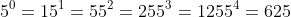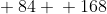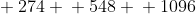0

## Wolfram|Alpha Factorial Trick...Improved!

Published on Thursday, December 13, 2012 in , , ,I was recently playing around with the Wolfram|Alpha Factorial Trick I wrote up a year ago, and I made a discovery that makes the feat much simpler!

The factorial of a number is the number you get when you multiply a whole number times all the numbers less than itself down to minus 1. For example, 5 factorial = 5 × 4 × 3 × 2 × 1 = 120.

Naturally, the larger the number, the more multiples of 5 and 2 you're going to use, so factorial often have a large number of zeroes at the end, known as trailing zeroes.

In this feat, you're able to quickly and easily give the number of trailing zeroes for the factorial of any number from 1 to 1000!

Make sure to read the original post, and are familiar with the basics of the routine. You should also be familiar with the fact that an exclamation point is used in math to denote factorial, such as 5! = 5 factorial = 5 × 4 × 3 × 2 × 1 = 120.

This new approach involves doubling a number chosen by the audience 1 or more times, so you first need to know exactly how many times to double the chosen number. As long as you know your powers of 5, this part is easy:If the chosen number is at least 50 and less than 51, then you won't need to double it at all, and the answer will have no trailing zeroes.

If the chosen number is at least 51 and less than 52, then you'll only need to double the chosen number once. If the chosen number is at least 52 and less than 52, then you'll only need to double the chosen number 2 times. See the pattern?Even though you'll be writing doubled numbers down, you're going to need to perform the doubling in your head. You can learn how to multiply 2-digit numbers by any 1-digit number in your head via MathTutor video, if needed. They also have a similar video that teaches you how to apply the same technique to 3-digit and higher numbers.

Now, you're about to learn the surprising power of simple doubling. I'll use 16 as a starting example. 16 is between 51 and 52, so I'll only need to double it once, so I think 16 × 2 = 32, and write down 32.

All you need to do know is cover up the rightmost digit of the doubled number, and that's the number of trailing zeroes in the factorial! In our example, the rightmost digit is 2, so we cover that up, leaving the 3. That means that 16! should have 3 trailing zeroes, and Wolfram|Alpha confirms that here.

If we try that with 22, we note it's also between 51 and 52, so we'll only need to double it 1 time, as well. 22 × 2 = 44, and if we cover up that rightmost 4, that leaves us with 4. Sure enough, 22! has 4 trailing zeroes!

When we have to double the chosen number more than once, there's are additional steps to take, but they're similar to what you've already learned. Also, when doubling 2 or more times, writing the doubled numbers down becomes very handy.

Let's try the new approach with the number 42. It's between 52 and 53, so we'll have to double it twice.

The first doubling of 42 is 84, and 84 doubled is 168, so yo'd write down those two numbers:Just as before, you're going to cover up the rightmost digit of the first doubling (the 4 in 84). With the second doubling, you're going to cover up the rightmost 2 digits. In our example, we're covering up the 6 and the 8 in 168. With those numbers out of sight, you're left with 8 and 1:All you do now is add up those remaining numbers! 8 and 1 are 9, and 42! does indeed have 9 trailing zeroes.

Notice the pattern when using 2 or more doublings. With the first doubling, you're ignoring the rightmost digit, and with the second doubling, you're ignoring the rightmost 2 digits. For the 3rd doubling, you'd ignore the 3 rightmost digits, and so on.

As a final example, let's work through this process with 137. 137 is between 53 and 54, so we'll need to double it 3 times, like this:For the first, second, and third doublings, ignore the rightmost 1, 2, and 3 digits respectively, and add up the remaining numbers (in your head, not on paper):Checking with Wolfram|Alpha again, we verify that 137! has 33 trailing zeroes.

Why does this work? It works for the same reason as the trick for dividing by 5 that's taught in the 2nd video in the original post. Dividing by 5 is the same thing as multiplying by and dividing that result by 10, which is easier to do in our heads. Dividing by 52, then, is the same thing as multiplying by 22, then dividing it by 102. Dividing by 53, then, is the same thing as multiplying by 23, then dividing it by 103.

The doubling of each number, of course, is the multiplying by 2. The simple act of ignoring the rightmost digits in each doubling is the same as dividing the first number by 10, the second number by 100, and so on, and the rounding those numbers down.

137 ÷ 5 = 27.4, and rounding that down lets us know that there are 27 sets of 5 in 137. See how this is the same as doubling 137 and ignoring the rightmost digit?

Similarly, 137 ÷ 25 = 5.48, and rounding down gives 5 complete sets of 25 in 137. Finally 137 ÷ 125 = 1.096, so we know there's only 1 complete 125 in 137.

The doubling process simply allows us to multiply by 2, which is easier for most people, and the concept of “ignoring” digits is disguising the decimals and even the division by powers of 10!

When actually performing this, it's good to have a reason to justify why you're writing down a series of doubled numbers. I suggest showing someone Russian Peasant Multiplication first, as taught in my Power of 2 post. Since you already have 1 column of doubled numbers at the end of that (make sure you don't use the column of halved numbers), you can use it for the factorial feat you just learned.

It may seem a little strange at first, but once you get the hang of it, it's quick fun and easy.